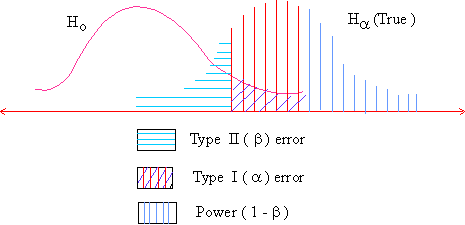Support the Monkey! Tell All your Friends and TeachersHome MonkeyNotes Printable Notes Digital Library Study Guides Study Smart Parents Tips College Planning Test Prep Fun Zone Help / FAQ How to Cite Request a New Title

8.10 Power Of A Hypothesis Test

The measure of how well the test of hypothesis is working, is called the 'power of the test'. In hypothesis testing both a ( the probability of Type-I error) and b (the probability of Type II error) should be small.

Type I error occurs when we reject Ho which is true and a (the significance level of the test) is the probability of making type - I error. Once the significance level is fixed, nothing can be done about a. Type II error occurs when we accept Ho which is false. The probability of type II error is b. The smaller the value of b, the better is the test. Alternately ( 1 - b ) i.e. the probability of rejecting Ho when it is false should be as large as possible.

Rejecting Ho when it is false is exactly what a good test must do. A high value of (1 - b ), mostly close to 1, means that the test is working in high gear. A low value of (1 - b ), mostly close 0, means that the test is working poorly.

Thus (1 - b ) is the measure of the power of the test. If we plot the values of ( 1 - b ) for each value of m f2 for which Ha is true, the resulting curve is known as a power curve.Your browser does not support the IFRAME tag.

You can see from the figure given below that the power is simply ( 1 - b ). In testing of a hypothesis high power is desirable. As we know that the Type I error or a is usually set in advance by a researcher but the Type II error or b for a given test is harder to know as it requires estimation of the distribution of Ha, which is unknown in most of the cases. So like b, the power can be difficult to estimate accurately, but increasing the sample size n, we can increase the power of the test.Index

8.1 Population
8.2 Sample
8.3 Parameters and Statistic
8.4 Sampling Distribution
8.5 Sampling Error
8.6 Central Limit Theorem
8.7 Critical Region
8.8 Testing of Hypothesis
8.9 Errors in Tesitng of Hypothesis
8.10 Power Of A Hypothesis Test
8.11 Sampling of Variables
8.12 Sampling of Attributes
8.13 Estimation
8.14 Testing the Difference Between Means
8.15 Test for Difference Between Proportions
8.16 Two Tailed and one Tailed Tests
8.17 Test of Significance for Small Samples
8.18 Students t-distribution
8.19 Distribution of 't' for Comparison of Two Samples Means Independent Samples
8.20 Testing Difference Between Mens of Two Samples Dependent Samples or Matched Paired Observations
8.21 Chi-Square
8.22 Sampling Theory of Correlation
8.23 Sampling Theory of Regression

Chapter 1Search: All Products Books Popular Music Classical Music Video DVD Toys & Games Electronics Software Tools & Hardware Outdoor Living Kitchen & Housewares Camera & Photo Cell Phones Keywords: